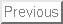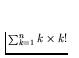# Computer Science 547 - Discrete Mathematics Prof. Steven Skiena Spring 1999

Homework 1
Due Tuesday, February 2, 1999

Each of the problems should be solved on a separate sheet of paper to facilitate grading. Limit the solution of each problem to one sheet of paper. Staple all these sheets together! Please don't wait until the last minute to look at the problems.

Do the following exercises in Graham, Knuth, and Patashnik:

1.
Warmup exercises of chapter 1: 1-7. (DO NOT TURN IN)

2.
Problem 1.8.

3.
Problem 1.16.

4.
Warmup exercises of chapter 2: 1-7. (DO NOT TURN IN)

5.
Problem 2.11.

6.
Problem 2.22.

7.
Problem 2.23a.

8.
Given a convex n-gon what is the maximum number of regions its interior can be divided into by diagonals connecting its vertices. A triangle defines one region, a square with its two diagonals defines four regions, and a pentagon with its five diagonals defines eleven regions.

a. Formulate a recurrence for the maximum number of regions.

b. Solve that recurrence.

9.
Find and prove a closed form formula for: